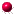XMM-Newton Science Analysis System

esensmap (esensmap-4.0) [xmmsas_20230412_1735-21.0.0]

# Description

esensmap creates a sensitivity map, providing upper limits to detect a point source over given background. In each image pixel, it includes the vignetting corrected source count rate (cts s) that corresponds to the minimum detection likelihood as specified in the parameter mlmin. From version 4.0 on, energy conversion factors (ECFsin units ofcts cmerg) are accepted via the parameter ecf to produce sensitivity maps in flux units (erg cms). The task parameter outunit determines whether to write count-rate maps (outunit=rate), flux maps (outunit=flux), or both (outunit=both).

eboxdetect-like Poisson mode

The task may either be called for individual energy bands or combinations of energy bands and instruments. The upper limits are derived by assuming Poissonian count statistics in each 33 pixel detection cell, using the exposure and background values read from the input images. It is assumed that 30 % of the PSF fall into the detection cell. Likelihoods are calculated as a function of raw source and background counts via the incomplete gamma function as described for eboxdetect. This simple upper limit calculation is meant as a reference point for a more detailed assessment of the sensitivity of the detection process to be determined through Monte Carlo simulations.

In the case of multiple input energy bands, the upper limits are calculated for the combined images. I.e. they refer to the detection sensitivity which would be achieved by adding up the photons observed in the individual bands (not identical to the sensitivity in a combined source-detection fit). Pixels outside of the detection mask as well as pixels with zero exposure time are assigned a sensitivity value of –1.0. This also holds for pixels where – potentially arising from a failure of the spline fit in the esplinemap backgound task – zero or negative values occur in the input background maps.

emldetect-like DeltaC mode

Version 4.0 introduces a DeltaC approach that is based on emldetect-like Cash statistic. It can be activated via the task parameter statistic=deltac and employs a likelihood test comparing the detection likelihood of background plus source emission with pure background emission. The log-likelihood ratio can be expressed via the Cash statisticas. This quantity, which follows adistribution approximately, is minimised in the emldetect source fit. Free parameters of this fit are the count rate in each input image, the source position, and, optionally, the source extent. The total detection likelihood is derived from the sum of the individualvalues (for more details, see for example Rosen et al. 2016, A&A 590, A1; Traulsen et al. 2019, A&A 624, A77).

The new esensmap mode adapts a simplified version of this emldetect approach, which was developed in the EU funded ARCHESproject. It uses background and exposure map(s) of an observation to determine the limiting count rate at a given total detection likelihood (parameter mlmin) in each of the exposed pixels, which are supplied as detection mask(s). If data of several instruments and/or energy bands are provided, the output sensitivity map gives the combined all-EPIC broad-band limits fordegrees of freedom, whereis the number of input images plus 3 (for source position and extent), similar to the combined fit performed by emldetect. To compute, the point-spread function (PSF) in each image pixel is interpolated from a grid of PSFs spanning different off-axis angles across the field of view. The parameter doff controls the off-axis steps. It defaults to 12 arcsec. Mid-band energies, at which the PSFs are obtained, are to be provided via the parameter energies. Parameter ecut controls the half size of the box within the PSF is evaluated, by default 15 arcsec (cf. the emldetect parameter ecut).

Since the EPIC PSFs are essentially rotationally symmetric, the azimuthal dependence of the PSF shapes (i.e., their orientation angle) is ignored for the sake of computational efficiency. This approximation leads to deviations from the true sensitivity of the order of several percent at the detector edges, where the PSFs are not covered completely (i.e. where the mask fraction is significantly smaller than one). Sensitivity values in these areas come with larger uncertainties anyway, as the source detection process itself.

XMM-Newton SOC -- 2023-04-16